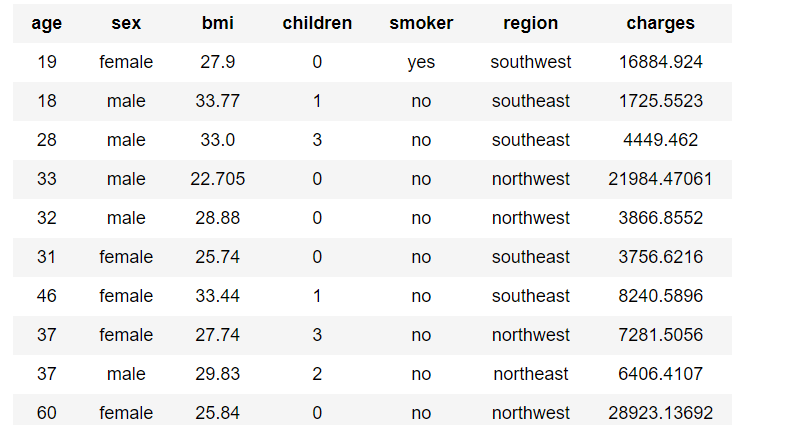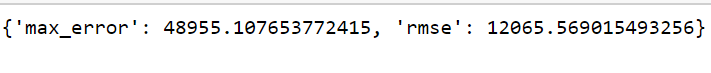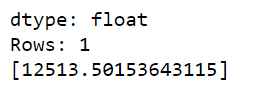# Linear Regression using Turicreate

Linear Regression is a method or approach for Supervised Learning.Supervised Learning takes the historical or past data and then train the model and predict the things according to the past results.Linear Regression comes from the word ‘Linear’ and ‘Regression’.Regression concept deals with predicting the future using the past data.Linear means the able to represented by a straight line on graph.Linear Regression has two things one independent variable and other dependent variable and Linear Regression is a relationship between the two.

In this article, we are going to learn about how we can implement Linear Regression with the help of Turicreate. Turicreate is Library in Python which helps the beginners to learn and implement Machine Learning Algorithm easily as well as efficiently.

Step 1: Importing the Turicreate Library

 `import` `turicreate as tc `

 `""" ` `The good thing about Turicreate is that we don't have ` `import any other library for data loading.Turicreate  ` `itself can load data with the help of it's SFrame  ` `Data Structure ` `"""` `data_sets ``=` `tc.SFrame(``"data.csv"``) `

Step 3: Exploring the data

 `# It will dispay the first few Lines of the data ` `data.head() `

Output:datasets first few lines

Step 4: Make a Linear Regression model .

 `# We will have a target variable that stores the thing ` `# to the predicted and feature is the list of elements ` `# which we will take for making the model. ` `model ``=` `tc.linear_regression.create( ` `    ``data, target ``=``"charges"``, features ``=``[``'region'``]) `

Step 5: Now evaluate the model

 `# this will tell us about the max error and  ` `# the rmse (root mean squared error) ` `model.evaluate(data) `

Output:Max error and Rmse

Step 6: Now predicting the charges according to the B.M.I of person

 `# this variable will be containing the data ` `# of person having the bmi 27.9 ` `bmi_person ``=` `data[data[``'bmi'``]``=``=` `27.9``] ` `# it will predict the charges for the person with bmi 27.9 ` `model.predict(bmi_person) `

Output:The Prediction of the charges

Attention geek! Strengthen your foundations with the Python Programming Foundation Course and learn the basics.

To begin with, your interview preparations Enhance your Data Structures concepts with the Python DS Course.

My Personal Notes arrow_drop_upIf you like GeeksforGeeks and would like to contribute, you can also write an article using contribute.geeksforgeeks.org or mail your article to contribute@geeksforgeeks.org. See your article appearing on the GeeksforGeeks main page and help other Geeks.

Please Improve this article if you find anything incorrect by clicking on the "Improve Article" button below.

Article Tags :
Practice Tags :

Be the First to upvote.

Please write to us at contribute@geeksforgeeks.org to report any issue with the above content.